# How to Calculate Weekly Average by Formula in Excel

In our daily life we often create tables for recording the money we spend for lunch or dinner or something else per date. This can help us know the total cost or average cost per date or other unit clearly via some statistic related functions in excel, and then we can cut back on unnecessary expenses and save our money.

Normally, one method for statistic in excel is creating pivot table. we can create a pivot table to auto calculate the total cost or average cost base on the values recorded in original table, and we can do filter by date or month or quarter to show different average cost for different period. Details see below screenshot.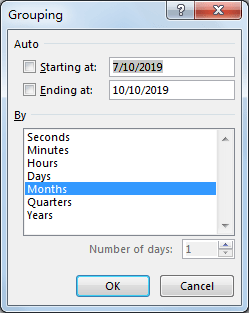Obviously, Week is not listed in default grouping date list.

If you want to know the method for counting average data by week (without using pivot table), you can read below article, it can help you to solve your question.

## Calculate Weekly Average by Formula in Excel

In Pre-Condition:

Prepare a simple table for demonstration. See screenshot below. You can see that we spend 10 or 5 or other values for lunch on different dates, some dates are included in one week. We want to count the weekly average for spend.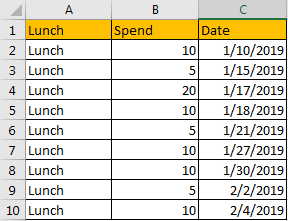Step 1: Create another table with three columns Week No., Year and Average. This table is used for recording the week number, year and the weekly average for launch spend.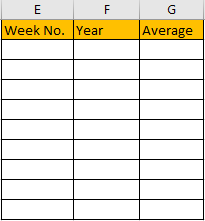Step 2: In E2, enter the formula =WEEKNUM(C2,2).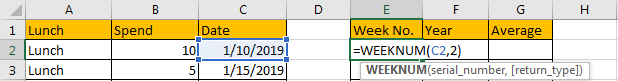For function WEEKNUM, we set the second parameter as 2, that means week begins on Monday.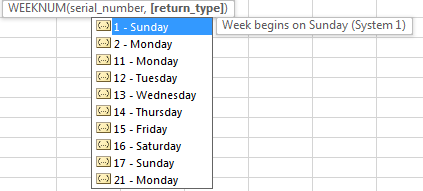Step 3: Click Enter to get returned value. We get 2 in this case. That means the date belongs to the second week in year 2019.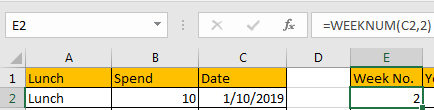Step 4: In F2, enter the formula =Year(C2). We can get Year 2019.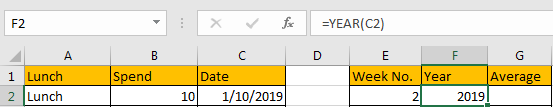Step 5: In G2, enter the formula =IF(AND(E2=E1,F2=F1),””,AVERAGEIFS(\$B\$2:\$B\$10,\$E\$2:\$E\$10,E2,\$F\$2:\$F\$10,F2)). Then we get the returned value 10.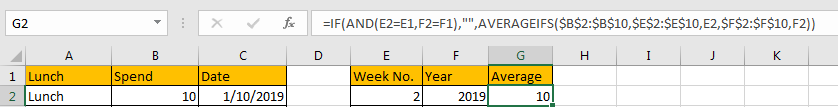Comment: In this formula, we use AVERAGEIFS function to calculate the average by specific criteria, and use IF function to classify dates by week and then get according average. If (E2=E1,F2=F1) is true, then we get blank value, otherwise we will get the weekly average.

Step 6: Drag the fill handle to fill the following cells in the table. We get weekly average values for all weeks.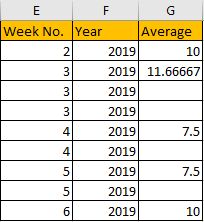Comment: If you want to ignore Year, you can just enter the formula =AVERAGEIFS(\$B\$2:\$B\$10,\$E\$2:\$E\$10,E2). Then we can get below table. You can depend on your requirement to determine whether add or remove criteria.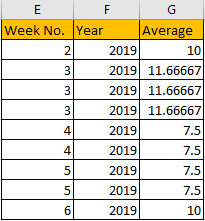### Related Functions

• Excel WEEKNUM function
The Excel WEEKNUM function returns the week number of a specific date, and the returned value is ranging from 1 to 53.The syntax of the WEEKNUM function is as below:=WEEKNUM (serial_number,[return_type])…
• Excel IF function
The Excel IF function perform a logical test to return one value if the condition is TRUE and return another value if the condition is FALSE. The IF function is a build-in function in Microsoft Excel and it is categorized as a Logical Function.The syntax of the IF function is as below:= IF (condition, [true_value], [false_value])….
• Excel AND function
The Excel AND function returns TRUE if all of arguments are TRUE, and it returns FALSE if any of arguments are FALSE.The syntax of the AND function is as below:= AND (condition1,[condition2],…)…

Related Posts

Check If a Cell is Blank or Empty

The article demonstrates how to check if a range of cells is blank or empty by ISBLANK and other functions to recognize empty cells in Excel and take appropriate action based on their status. There are several circumstances in which ...

Abbreviate Names Or Words in Excel

As an MS Excel user, you might have come across a task where you need to abbreviate different names or words, and there are also possibilities that you might have done this task manually by assuming that there isn't any ...

Extract or Filter on The Top N Values with Criteria

Suppose that you are in a situation where you need to filter out the top n values from the list having few values with specific criteria, or you can say that with a particular condition, and I am also pretty ...

Extract matching values From Two Lists

Suppose that you are working with two lists containing few values, and you want to extract the matching values from those two lists into another separate list. You might prefer to manually extract the matching values from the two lists, ...

Extract Multiple Match Values into Separate Columns

If you have a few values/items in the excel sheet and you are thinking that with the aid of the “VlOOKUP” function you can look for a specific value, extract it and then put the matching item into the separate ...

VLOOKUP Formula | Faster Trick with 2 VLOOKUPS

This post will guide you how to use 2 VLOOKUPS function to looking up data entries from a given range of cells in Microsoft Excel. VLOOKUP with 2 lookups can be faster than a single VLOOKUP in certain scenarios. The ...

Extract all the matches with helper Column

With Excel's powerful functions IF, INDEX, and MATCH, we can find exactly what you're looking for with a few clicks of the mouse. This step-by-step tutorial will show how easy it is to extract data using these tools and more! ...

If Cell is This Value or That Value

IF function is frequently used in Excel worksheet to return you expect “true value” or “false value” based on the result of logical test. If you want to see if a cell is A or B, and if one of ...

If Value is Greater Than A Certain Value

IF function is frequently used in Excel worksheet to return you expect “true value” or “false value” based on the logical test result. If you want to see if a value in one cell is greater than a specific value, ...

If Cell is Not Blank

IF function is frequently used in Excel worksheet to return you expect “true value” or “false value” based on the result of created logical test. If you want to see if a cell is blank or not, and leave some ...

Sidebar